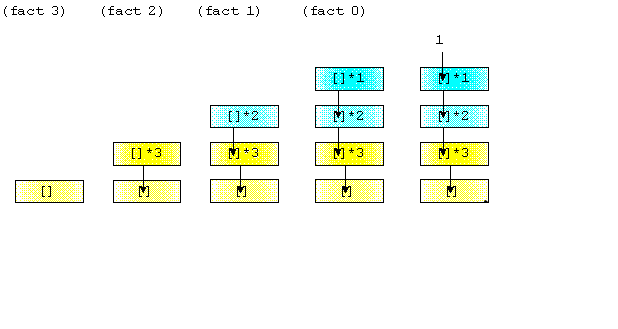# Tail Recursion

## Introduction

A recursive function is called Tail recursive if the recursive call is the last thing done by the function. There is no need to keep records of the previous state. The tail recursion uses the recursive function as the last statement of the function. So in it, nothing is left to do after coming back from the recursive call.

## Explanation with Code

### Printing the number from N to 1.

``````public class tail_rec {
public static void printNum(int n){
if(n==0){
return;
}
System.out.print(n+" ");
printNum(n-1);
}
public static void main(String[] args){
printNum(10);
}
}
``````

As of now, see the above example of calling the function printNum as we recall the concept of the recursion in which all the values are gets stored in the form of the stack in each function call and at the base condition what we return for value for problem-solving that helps to calculate, the overall solution with that. Still, in Tail recursion, we don't require any previous value as such, for overall calculation of solution of the problem.

As seeing the problem for printing the number from n to 1 in a recursive way for Tail recursion, types for when we pass the integer value of 10 to the function then we see that we have the base over the n==0, it's just return nothing, and after that, no call such is there, which help to find the overall solution, as per the question we see there at every call we are printing the value which is the main task. We have not used store value for further use. In the recursion call, it gets inserted in the recursion stack, but we are not using it, as no such call is further made after the last call of the function. This is a way of Tail recursion.

### Factorial of a number

``````public class tail_rec {
public static int printfact(int n,int a){
if(n==0||n==1){
return a;
}
return printfact(n-1,n*a);
}
public static void main(String[] args){
System.out.print(printfact(10,1));
}
}``````

As thinking about the above code for the factorial of a number as we see the things as such that, when we are making the recursion call, then a recursion stack is created and at first main function call is started, and on moving at each call by passing the parameter to printfact of an of a given number to a function declared, then we also pass an additional parameter as an “a” because we are updating the value of factorial of a number to given value from the higher-order number to lower if we pass 10, then.

1. For first, it's a 10*1 for the first pass, now n=9 for the next pass.For Second, it's a 9*10, now n=8 for the next pass.
2. For third, it’s an 8*90 and now n=7 for the next pass.
3. …….. It continues till the base condition is reached, i.e., n=0, and returns a.

In this way, we repeat the step, and at last, we directly return the value of “a”,  which

Having all the stored multiples till the base condition is reached, we have to not go to the recursion stack again and again for further calculation, so we save our time here also.

E.g., until, Now take another example by passing n=3 for calculation.,Source: Factorial

``````printfact(3, 1)
return printfact (2,  1 * 3 ) //n == 3
return printfact (1,  3 *2 ) //n == 2
return 6  //n == 1``````

So, we can see that each time the printfact function is called in our example, a new value for the current_printfact variable is passed into the printfact function. The function is basically updating the current_printfact variable with each call to the function. We can maintain the current factorial value because this function accepts two parameters, not just one like our normal recursive printfact function above.

## Difference between Tail Recursion vs Normal Recursion

In the traditional recursion or normal recursion, we have to use the recursion stack after the base condition, but in the Tail recursion, we have nothing to do with the recursion stack after the base condition encounter.

### For the tail recursion

Hereafter the base condition encounter, we just return the value of a, nothing to do after that.

Explanation:-### For the Normal recursion

Hereafter the base condition, we return the value 1, then we use the recursion stack to calculate the value of factorial of 5.

Explanation:-## FAQ's

1). What is a different type of Recursion?

There are two types of recursion:-

1. Tail Recursion

2. Normal Recursion

2). How is Tail Recursion better than the Normal recursion?

The tail recursion is better than Normal recursion. There is no task left after the recursive call; it can be bypassed, more manageable for the compiler to optimize the code. When one function is called, its address is stored inside the stack. So if it is tail recursion, then storing addresses into the stack is not needed.

## Key TakeAway

In this blog, we learned about the concept of Tail recursion and its benefits of not using the recursion stack, again for finding a solution how the Tail recursion concept works are understood with the help of two examples along with the code. We compare the concept of usual recursion to Tail recursion. Tail recursion helps in the storage part, as we do not need a recursion stack, as function call on calling is summing the solution for the problem.